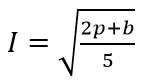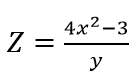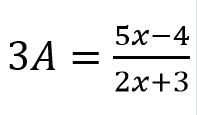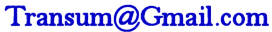# Changing The Subject

## Rearrange a formula in order to find a new subject in this self marking exercise.

##### Level 1Level 2Level 3Level 4Level 5Level 6DescriptionHelpMore Algebra

This is level 6: finding the unknown which is not the subject of a formula You can earn a trophy if you get at least 9 correct and you do this activity online.

 If a = 2b + c, find c when a=15 and b=5 $$c=$$If a = 2b + c, find b when a=16 and c=8 $$b=$$If e = 3(f + g), find g when e=18 and f=3 $$g=$$If e = 4(f + g), find f when e=15 and g=8 $$f=$$If m = n² + 4p, find p when m=24 and n=24 $$p=$$If m = n² + 9p, find n when m=135 and p=6 $$n=$$If w = (u - 2v)², find v when w=4 and u=-16 $$v=$$If 4j = (k - 8h)², find k when j=9 and h=-5 $$k=$$If a = c - ¼b², find c when a=-8 and b=-28 $$c=$$find p when: I=6 and b=3 $$p=$$find x when: Z=19.3 and y=10 $$x=$$find x when: A=1  $$x=$$Check

This is Changing The Subject level 6. You can also try:
Level 1 Level 2 Level 3 Level 4 Level 5

## Instructions

Try your best to answer the questions above. Type your answers into the boxes provided leaving no spaces. As you work through the exercise regularly click the "check" button. If you have any wrong answers, do your best to do corrections but if there is anything you don't understand, please ask your teacher for help.

When you have got all of the questions correct you may want to print out this page and paste it into your exercise book. If you keep your work in an ePortfolio you could take a screen shot of your answers and paste that into your Maths file.

## Transum.org

This web site contains over a thousand free mathematical activities for teachers and pupils. Click here to go to the main page which links to all of the resources available.

Please contact me if you have any suggestions or questions.## More Activities:

Mathematicians are not the people who find Maths easy; they are the people who enjoy how mystifying, puzzling and hard it is. Are you a mathematician?

Comment recorded on the 10 September 'Starter of the Day' page by Carol, Sheffield PArk Academy:

"3 NQTs in the department, I'm new subject leader in this new academy - Starters R Great!! Lovely resource for stimulating learning and getting eveyone off to a good start. Thank you!!"

Comment recorded on the 9 October 'Starter of the Day' page by Mr Jones, Wales:

"I think that having a starter of the day helps improve maths in general. My pupils say they love them!!!"

#### PentransumAnswer multiple choice questions about basic mathematical ideas. If you get a number of questions correct you will be invited to post a question of your own. The bank of questions grows larger every day.

There are answers to this exercise but they are available in this space to teachers, tutors and parents who have logged in to their Transum subscription on this computer.

A Transum subscription unlocks the answers to the online exercises, quizzes and puzzles. It also provides the teacher with access to quality external links on each of the Transum Topic pages and the facility to add to the collection themselves.

Subscribers can manage class lists, lesson plans and assessment data in the Class Admin application and have access to reports of the Transum Trophies earned by class members.

If you would like to enjoy ad-free access to the thousands of Transum resources, receive our monthly newsletter, unlock the printable worksheets and see our Maths Lesson Finishers then sign up for a subscription now:

Subscribe

## Go Maths

Learning and understanding Mathematics, at every level, requires learner engagement. Mathematics is not a spectator sport. Sometimes traditional teaching fails to actively involve students. One way to address the problem is through the use of interactive activities and this web site provides many of those. The Go Maths page is an alphabetical list of free activities designed for students in Secondary/High school.

## Maths Map

Are you looking for something specific? An exercise to supplement the topic you are studying at school at the moment perhaps. Navigate using our Maths Map to find exercises, puzzles and Maths lesson starters grouped by topic.

## Teachers

If you found this activity useful don't forget to record it in your scheme of work or learning management system. The short URL, ready to be copied and pasted, is as follows:

Do you have any comments? It is always useful to receive feedback and helps make this free resource even more useful for those learning Mathematics anywhere in the world. Click here to enter your comments.For All:

© Transum Mathematics :: This activity can be found online at:
www.transum.org/software/SW/Starter_of_the_day/Students/Changing_The_Subject.asp?Level=6

## Description of Levels

CloseLevel 1 - Formulas which can be rearranged by adding or subtracting terms from both sides

Example: Make e the subject of the formula d = e - f

Level 2 - Formulas which can be rearranged by multiplying or dividing both sides by a value

Example: Rearrange the formula n = mp

Level 3 - Formulas which can be rearranged by adding, subtracting, multiplying or dividing both sides by a value

Example: Rearrange the formula b = a + cd

Level 4 - Formulas including brackets or expressions in the numerator or denominator of a fraction

Example: Rearrange the formula p = s(t + 2)

Level 5 - Formulas including squares or square roots

Example: Rearrange the formula d² = 2a + 1

Level 6 - Finding the unknown which is not the subject of a formula

Example: If m = n² + 2p, find p when m=8 and n=10

Answers to this exercise are available lower down this page when you are logged in to your Transum account. If you don’t yet have a Transum subscription one can be very quickly set up if you are a teacher, tutor or parent.

## Example

This video provides help on 'changing the subject' and is from Corbettmaths.

Don't wait until you have finished the exercise before you click on the 'Check' button. Click it often as you work through the questions to see if you are answering them correctly.

Answers to this exercise are available lower down this page when you are logged in to your Transum account. If you don’t yet have a Transum subscription one can be very quickly set up if you are a teacher, tutor or parent.

Close